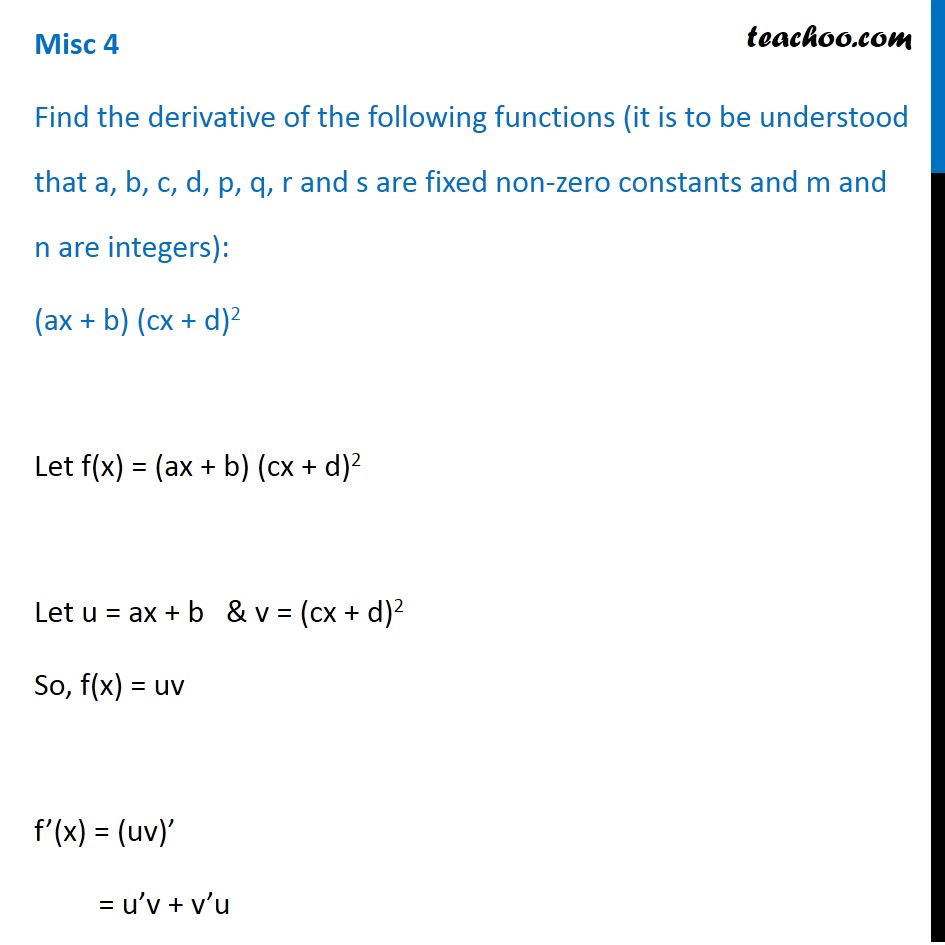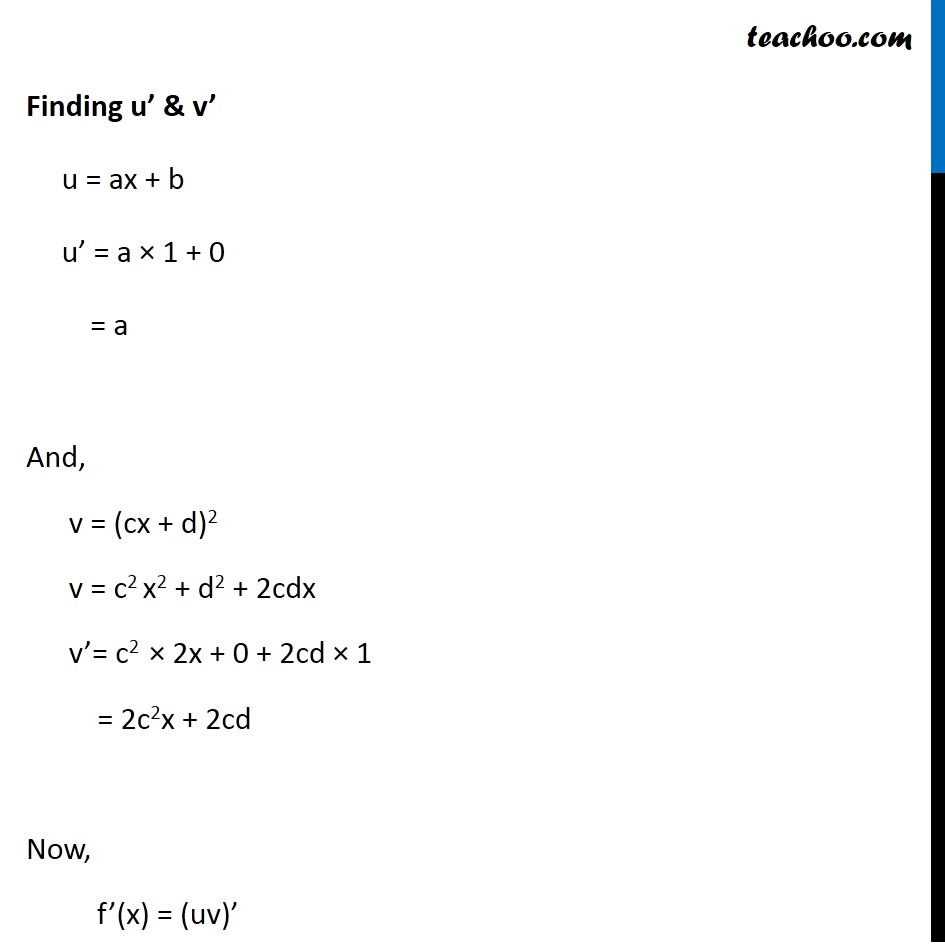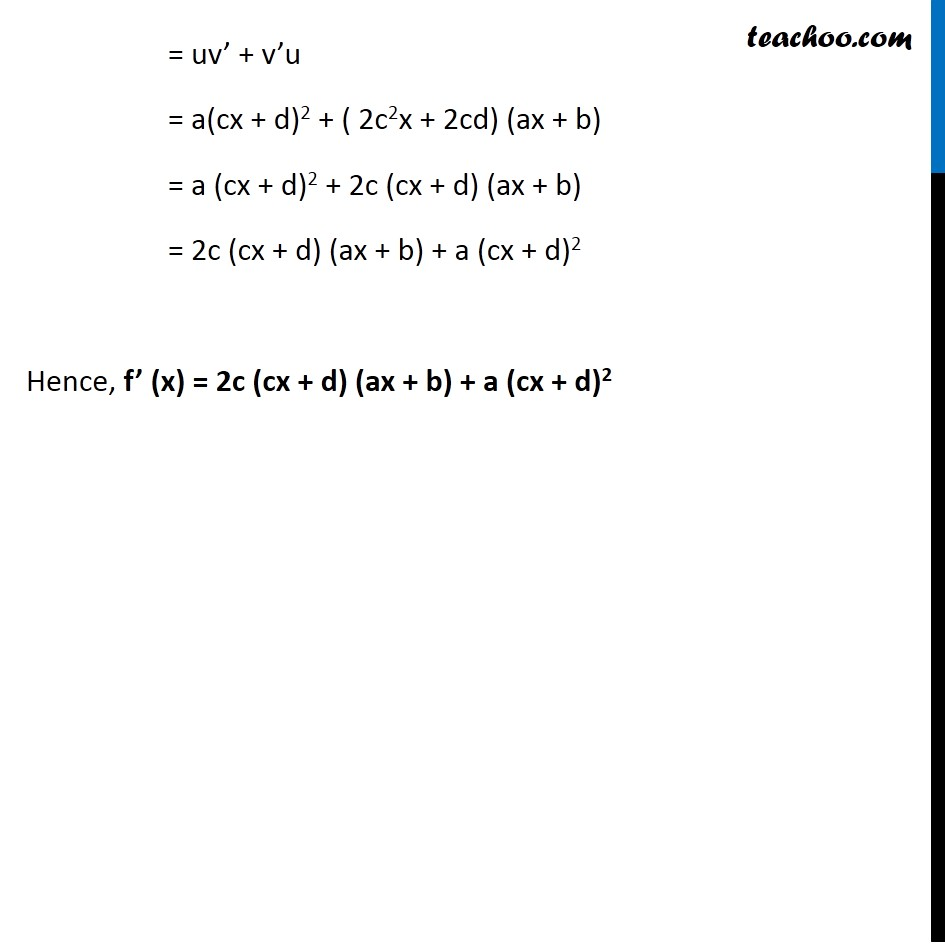Miscellaneous

Chapter 12 Class 11 Limits and Derivatives
Serial order wiseLearn in your speed, with individual attention - Teachoo Maths 1-on-1 Class

### Transcript

Misc 4 Find the derivative of the following functions (it is to be understood that a, b, c, d, p, q, r and s are fixed non-zero constants and m and n are integers): (ax + b) (cx + d)2 Let f(x) = (ax + b) (cx + d)2 Let u = ax + b & v = (cx + d)2 So, f(x) = uv f’(x) = (uv)’ = u’v + v’u Finding u’ & v’ u = ax + b u’ = a × 1 + 0 = a And, v = (cx + d)2 v = c2 x2 + d2 + 2cdx v’= c2 × 2x + 0 + 2cd × 1 = 2c2x + 2cd Now, f’(x) = (uv)’ = uv’ + v’u = a(cx + d)2 + ( 2c2x + 2cd) (ax + b) = a (cx + d)2 + 2c (cx + d) (ax + b) = 2c (cx + d) (ax + b) + a (cx + d)2 Hence, f’ (x) = 2c (cx + d) (ax + b) + a (cx + d)2